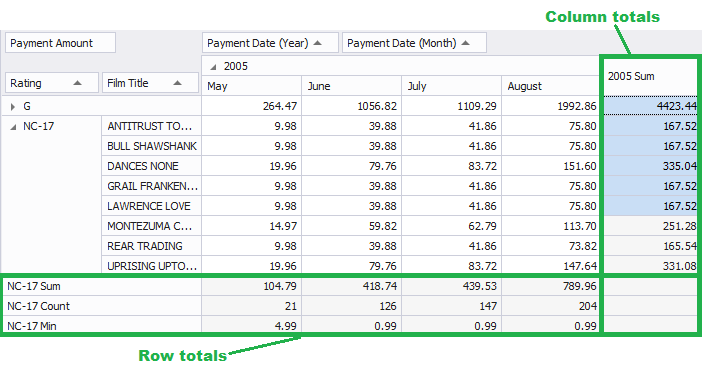# Totals

Row Totals are rows containing a calculated sum of row field values. Column totals are columns containing a calculated sum of column field values.

Totals are calculated based on selected summary functions and displayed in the Data area highlighted with the gray color. The default function calculates the sum of all values. You can cancel the default function or, quite the contrary, add others.